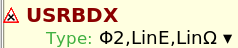# Discrepancy between USERDUMP and USRBDX

Dear FLUKA experts,
I was working on simulation where neutrons impact on a lead target. My goal was to count how many neutrons exits from the lead.

In order to do this I used both the USRBDX card and wrote a custom mgdraw.f routine using the BDXDRAW entry to save the neutrons that exit from the lead.

I did a comparison and I found that the number of neutrons that exit the lead given by the USRBDX card is not equal to the number I obtain from USERDUMP.

The results I got are:

phi1 phi2
Usrbdx results (_sum.lis) 0.3314297 * 2M = 662 859.4 0.3980420 * 2M = 796 084
Usrbdx results (_tab.lis) 664 070 797 788
Userdump results (.txt → root) 453 776.0 515 677.0

So:

• For the first row I just take the number quoted on the _sum.lis produced by USRBDX and multiply it by the total number of primaries simulated
• For the second row I wrote a code that build an histogram from the _tab.lis file, compute the integral of the histogram (taking into account that every bin content needs to be multiplied by its bin width) and last multiply it by the surface area (given in USRBDX) and the total number of primaries
``````    TH1D *histo = new TH1D("spectrum", "Neutron Spectrum; E (GeV); Neutrons/GeV/cm^{2}/pr", lowBins.size()-1, lowBins.data());
for(int i = 0; i<contents.size(); i++){
histo->SetBinContent(i+1, contents[i]);                 // 1/pr/cmq/GeV
histo->SetBinError(i+1, errors[i]*contents[i]/100);     //from % to abs
}

TCanvas *c = new TCanvas("canvas", "canvas", 500, 500);
histo->Draw("histe");
cout << histo->Integral("width") * 2000000 * 192 << endl;
``````
• For the third row I convert the .txt file produced by the mgdraw routing in a .root file (because it is easier for me to analyze the results of several simulations coming from different jobs at the same time). Then I build an histogram from the data inside the .root file (for example I build an histogram of the total energy from the column “ETot” of my RDataFrame). Then i calculate the integral, here I don’t normalize beacuse I already have particles.
``````import ROOT

Events = ROOT.RDataFrame("Events", "root_files/*.root")

h = Events.Histo1D("ETot")
integral = h.Integral()
print(integral)

c = ROOT.TCanvas("c", "c", 500, 500)
h.Draw()
c.Update()
``````

Now, I think that the first 2 are different because of the precision of the calculation.
But for the last one I don’t know for the last one.
I am surely missing something or doing something wrong, can you help me understand why the two results are different?

Here are the file that I used for the simulation:
ftelos.f (2.1 KB)
mgdraw.f (6.0 KB)

Here are the file that I use to analyze the USERDUMP results:
to_root.py (2.4 KB) (works with ROOT 6.28/02)
analyze.py (394 Bytes)

Hi Antonino,

In your USRBDX card you selected:This is a fluence estimator. However you said that:

My goal was to count how many neutrons exits from the lead.

In this case, a current estimator would be more appropriate. In that case, your results should match the one you get with your user routine, which effectively counts the particle flowing through the regions, without considering the angle.

To further reads, you can see pages 2-3 of the beginner course dedicated to differential spectra: https://indico.cern.ch/event/1123370/contributions/4716029/attachments/2446126/4191571/10_Scoring_II_2022_ULB.pdf

1 Like

Hi Daniele,
now the results match!
Thanks a lot!